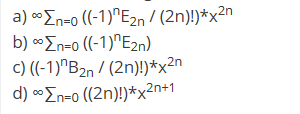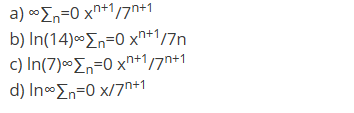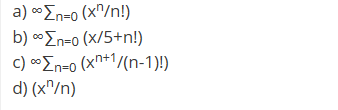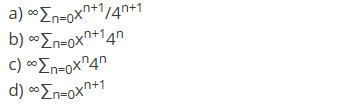# Welcome to the Discrete Probability MCQs Page

Dive deep into the fascinating world of Discrete Probability with our comprehensive set of Multiple-Choice Questions (MCQs). This page is dedicated to exploring the fundamental concepts and intricacies of Discrete Probability, a crucial aspect of Discrete Mathematics. In this section, you will encounter a diverse range of MCQs that cover various aspects of Discrete Probability, from the basic principles to advanced topics. Each question is thoughtfully crafted to challenge your knowledge and deepen your understanding of this critical subcategory within Discrete Mathematics.

Check out the MCQs below to embark on an enriching journey through Discrete Probability. Test your knowledge, expand your horizons, and solidify your grasp on this vital area of Discrete Mathematics.

Note: Each MCQ comes with multiple answer choices. Select the most appropriate option and test your understanding of Discrete Probability. You can click on an option to test your knowledge before viewing the solution for a MCQ. Happy learning!

### Discrete Probability MCQs | Page 1 of 10

Q1.
sec(x) has a trigonometric series that is given by _______a.

a

b.

b

c.

c

d.

d

Q2.
Determine a power series representation for the function g(x)=ln(7−x).a.

a

b.

b

c.

c

d.

d

Q3.
An example of Maclaurin series is _______a.

a

b.

b

c.

c

d.

d

Q4.
Find the power series representation for the function f(x)=x/4−x.a.

a

b.

b

c.

c

d.

d

Q5.
Neha has 4 yellow t-shirts, 6 black t-shirts, and 2 blue t-shirts to choose from for her outfit today. She chooses a t-shirt randomly with each t-shirt equally likely to be chosen. Find the probability that a black or blue t-shirt is chosen for the outfit.
Answer: (c).$$\frac{1}{2}$$
Q6.
If a fair 15-sided dice is rolled, then is the probability that the roll is an odd number or prime number or both?
Answer: (c).$$\frac{9}{20}$$
Q7.
There are a total of 50 distinct books on a shelf such as 20 math books, 16 physics books, and 14 chemistry books. Find is the probability of getting a book that is not a chemistry book or not a physics book.

d.

1

Q8.
A number is selected from the first 20 natural numbers. Find the probability that it would be divisible by 3 or 7?
Answer: (d).$$\frac{7}{20}$$
Q9.
There are 24 red marbles in a bag 68 marbles, and 8 of those marbles are both red and white striped. 27 marbles are white striped and of those marbles, the same 8 marbles would be both red and white striped). Find the probability of drawing out a marble from the bag that is either red or white striped.
Answer: (b).$$\frac{43}{68}$$
Q10.
If spinner has 3 equal sectors colored yellow, blue and red, then the probability of landing on red or yellow after spinning this spinner is _______
Answer: (a).$$\frac{2}{3}$$
Page 1 of 10

# Suggested Topics

Are you eager to expand your knowledge beyond Discrete Mathematics? We've curated a selection of related categories that you might find intriguing.

Click on the categories below to discover a wealth of MCQs and enrich your understanding of Computer Science. Happy exploring!